# PSEB 12th Class Physics Solutions Chapter 12 Atoms

Punjab State Board PSEB 12th Class Physics Book Solutions Chapter 12 Atoms Textbook Exercise Questions and Answers.

## PSEB Solutions for Class 12 Physics Chapter 12 Atoms

PSEB 12th Class Physics Guide Atoms Textbook Questions and Answers

Question 1.
Choose the correct alternative from the clues given at the end of the each statement:
(a) The size of the atom in Thomson’s model is ………………….. the atomic size in Rutherford’s model, (much greater than/no different from/much less than.)
(b) In the ground state of ………………………………… electrons are in stable equilibrium, while in …………………….. electrons always experience a net force. (Thomson’s model/Rutherford’s model.)
(c) A classical atom based on ……………………………. is doomed to collapse. (Thomson’s model/Rutherford’s model.)
(d) An atom has a nearly continuous mass distribution in a ………………………… but has a highly non-uniform mass distribution in …………………….. (Thomson’s model/Rutherford’s model.)
(e) The positively charged part of the atom possesses most of ………………………. the mass in ………………….. (Rutherford’s model/both the models.)
(a) The size of the atom in Thomson’s model is no different from the atomic size in Rutherford’s model.
(b) In the ground state of Thomson’s model, electrons are in stable equilibrium while, in Rutherford’s model, electrons always experience a net force.
(c) A classical atom based on Rutherford’s model is doomed to collapse.
(d) An atom has a nearly continuous mass distribution in Thomson’s model but has a highly non-uniform mass distribution in Rutherford’s model.
(e) The positively charged part of the atom possesses most of the mass in both the models.

Question 2.
Suppose you are given a chance to repeat the alpha-particle scattering experiment using a thin sheet of solid hydrogen in place of the gold foil. (Hydrogen is a solid at temperatures below 14 K.) What results do you expect?
The basic purpose of scattering experiment is not completed because solid hydrogen will be a much lighter target as compared to the alpha particle acting as a projectile. By using the conditions of elastic collisions, the hydrogen will move much faster as compared to alpha after the collision. We cannot determine the size of hydrogen nucleus.Question 3.
What is the shortest wavelength present in the Paschen series of spectral lines?
Rydberg’s formula is given as
$$\frac{h c}{\lambda}$$ = $$21.76 \times 10^{-19}\left[\frac{1}{n_{1}^{2}}-\frac{1}{n_{2}^{2}}\right]$$
where, h = Planck’s constant = 6.63 x 10-34 Js
c=Speed oflight=3 x 108 m/s (n1 and n2 are integers)
The shortest wavelength present in the Paschen series of the spectral lines
is given for values n1 = 3 and n2 = ∞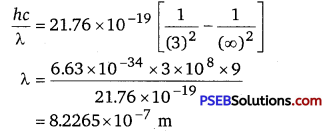= 822.65 nm

Question 4.
A difference of 2.3 eV separates two energy levels in an atom. What is the frequency of radiation emitted when the atom makes a transition from the upper level to the lower level?
According to Bohr’s postulate
E2 – E1 = hv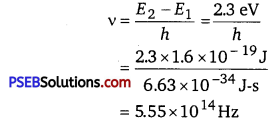Question 5.
The ground state energy of hydrogen atom is -13.6 eV. What are the kinetic and potential energies of the electron in this state?
Given, the ground state energy of hydrogen atom
E=-13.6eV
We know that,
Kinetic Energy, EK = -E = 13.6 eV
Potential Energy Ep = -2KE =-2 x 13.6 = -27.2eV

Question 6.
A hydrogen atom initially In the ground level absorbs a photon, which excites it to the n = 4 level. Determine the wavelength and frequency of photons.
The energy levels of H-atom are given by
En = $$-\frac{R h c}{n^{2}}$$
For given transition n1 =1, n2 = 4
∴ E1 = $$-\frac{R h c}{1^{2}}$$ ,E2= $$-\frac{R h c}{4^{2}}$$
∴ Energy of absorbed photon
ΔE=E2 -E1 =Rhc $$\left(\frac{1}{1^{2}}-\frac{1}{4^{2}}\right)$$
or
ΔE = $$\frac{15}{16}$$ Rhc ………………………….. (1)
∴ The wavelength of absorbed photon λ is given by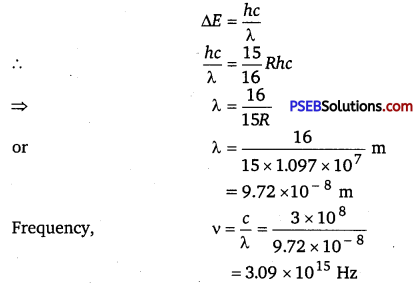Question 7.
(a) Using the Bohr’s model, calculate the speed of the electron in a hydrogen atom in the n =1, 2, and 3 levels.
(b) Calculate the orbital period in each of these levels.
(a) Let y1 be the orbital speed of the electron in a hydrogen atom in the ground state level, n1 =1.
For charge (e) of an electron, v1 is given by the relation,
v1 = $$\frac{e^{2}}{n_{1} 4 \pi \varepsilon_{0}\left(\frac{h}{2 \pi}\right)}=\frac{e^{2}}{2 \varepsilon_{0} h}$$
where, e=1.6 x 10-19 C
$$\varepsilon_{0}$$ = Permittivity of free space = 8.85 x 10-12 N-1 C2m2
h = Planck’s constant = 6.63 x 10-34 Js
∴ v1 = $$\frac{\left(1.6 \times 10^{-19}\right)^{2}}{2 \times 8.85 \times 10^{-12} \times 6.63 \times 10^{-34}}$$
= 0.0218 x 108 =2.18 x 106 m/s

For level n2 =2, we can write the relation for the corresponding orbital speed as
v2 = $$\frac{e^{2}}{n_{2} 2 \varepsilon_{0} h}$$ = $$\frac{\left(1.6 \times 10^{-19}\right)^{2}}{2 \times 2 \times 8.85 \times 10^{-12} \times 6.63 \times 10^{-34}}$$ = 1.09 x 106 m/s
And, for n3 =3, we can write the relation for the corresponding orbital speed as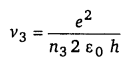Hence, the speed of the electron in a hydrogen atom in n = 1, n = 2 and n = 3 is 2.18 x 106 m/s,
1.09 x 106 m/s, 7.27 x 105 m/s respectively.

(b) Orbital period of electron is given by
T = $$\frac{2 \pi r}{v}$$
rn = $$\frac{n^{2} h^{2}}{4 \pi^{2} K m e^{2}}$$
∴ r1 = $$\frac{(1)^{2} \times\left(6.63 \times 10^{-34}\right)^{2}}{4 \times 9.87 \times\left(9 \times 10^{9}\right) \times 9 \times 10^{-31} \times\left(1.6 \times 10^{-19}\right)}$$
= 0.53 x 10-10 m
For n=1, T1 = $$\frac{2 \pi r_{1}}{v_{1}}$$
= $$\frac{2 \times 3.14 \times 0.53 \times 10^{-10}}{2.19 \times 10^{6}}$$ = 1.52 x 10-16s

For n = 2, radius rn = n2r1
∴ r2 =’22.r1 =4 x0.53 x 10-10
and velocity vn, = $$\frac{v_{1}}{n}$$
∴ v2 = $$\frac{v_{1}}{2}=\frac{2.19 \times 10^{6}}{2}$$
Time period T2 = $$\frac{2 \times 3.14 \times 4 \times 0.53 \times 10^{-10} \times 2}{2.19 \times 10^{6}}$$
=1216 x 10-15 s
For n=3,radius r3 =32,r1 =9r1 =9 x 0.53 x 10-10m and velocity v3 = $$\frac{v_{1}}{3}=\frac{2.19 \times 10^{6}}{3}$$ m/s
Time period T3 = $$\frac{2 \pi r_{3}}{v_{3}}=\frac{2 \times 3.14 \times 9 \times 0.53 \times 10^{-10} \times 3}{2.19 \times 10^{6}}$$ = 4.1 x 10-15 sQuestion 8.
The radius of the innermost electron orbit of a hydrogen atom is 5.3 x 10-11 m. What are the radii of the n = 2 and n = 3 orbits?
The radius of the innermost electron orbit of a hydrogen atom, r1 = 5.3 x 10-11 m.
Let r2 be the radius of the orbit at n = 2.
It is related to the radius of the innermost orbit as r2 = (n)2r1 = (2)2 x 5.3 x 10-11
= 4 x 5.3 x 10-11 = 2.12 x 10-10m
For n = 3, we can write the corresponding electron radius as
r3 =(n)2r1 = (3)2 x 5.3 x 10-11
n = 9 x 5.3 x 10-11 = 4.77 x 10-10m
Hence, the radii of an electron for n = 2 and n = 3 orbits are 2.12 x 10-10 m and 4.77 x 10-10 m respectively.

Question 9.
A 12.5 eV electron beam is used to bombard gaseous hydrogen at room temperature. What series of wavelengths will be emitted?
It is given that the energy of the electron beam used to bombard gaseous hydrogen at room temperature is 12.5 eV. Also, the energy of the gaseous hydrogen in its ground state at room temperature is -13.6 eV. When gaseous hydrogen is bombarded with an electron beam, the energy of the gaseous hydrogen becomes -13.6 + 12.5 eV i. e., -1.1 eV.

Orbital energy is related to orbit level (n) as
E = $$\frac{-13.6}{(n)^{2}}$$eV
For n=3, E = $$\frac{-13.6}{(3)^{2}}=\frac{-13.6}{9}$$ = -1.5 eV
This energy is approximately equal to the energy of gaseous hydrogen. it can be concluded that the electron has jumped from n I to n = 3 level.

During its de-excitation, the electrons can jump from n = 3 to n = 1 directly, which forms a line of the Lyman series of the hydrogen spectrum.
We have the relation for wave number for Lyman series as
$$\frac{1}{\lambda}=R_{y}\left(\frac{1}{1^{2}}-\frac{1}{n^{2}}\right)$$
where, Ry =Rydberg constant = 1.097 x 107 m-1,
λ = Wavelength of radiation emitted by the transition of the electron for
n =3,
We can obtain λ as
$$\frac{1}{\lambda}$$ = 1.097 x 107$$\left(\frac{1}{1^{2}}-\frac{1}{3^{2}}\right)$$
= 1.097 x 107 $$\left(1-\frac{1}{9}\right)$$ = 1.097 x 107x $$\frac{8}{9}$$

λ = $$\frac{9}{8 \times 1.097 \times 10^{7}}$$ = 102.55nm
If the electron jumps from n = 2 to n = 1, then the wavelength of the radiation is given as
$$\frac{1}{\lambda}$$ = 1.097 x 107 $$\left(\frac{1}{1^{2}}-\frac{1}{2^{2}}\right)$$
= 1.097 x 107$$\left(1-\frac{1}{4}\right)$$ = 1.097 x 107x $$\frac{3}{4}$$
λ = $$\frac{4}{1.097 \times 10^{7} \times 3}$$ = 121.54 nm

If the transition takes place from n = 3 to n = 2, then the wavelength of the radiation is given as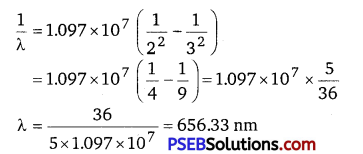This radiation corresponds to the Balmer series of the hydrogen spectrum. Hence, in Lyman series, two wavelengths i. e., 102.54 nm, and 121.55 nm are emitted. And in the Balmer series, one wavelength i. e., 656.33 nm is emitted.

Question 10.
In accordance with the Bohr’s model, find the quantum number that characterizes the earth’s revolution around the sun in an orbit of radius 1.5 x 1011 m with orbital speed 3 x 104 m/s. (Mass of earth = 6.0 x 1024 kg.)
Radius of the orbit of the Earth around the Sun, r = 1.5 x 1011 m
Orbital speed of the Earth, v = 3 x 104 m/s
Mass of the Earth, m = 6.0 x 1024 kg
According to Bohr’s model, angular momentum is quantized and given as
mvr = $$\frac{n h}{2 \pi}$$

where, h = Planck’s constant = 6.63 x 10-34 Js
n = Quantum number
∴ n = $$\frac{m v r 2 \pi}{h}$$
= $$\frac{2 \pi \times 6 \times 10^{24} \times 3 \times 10^{4} \times 1.5 \times 10^{11}}{6.63 \times 10^{-34}}$$ = 25.61 x 1073 = 2.6 x 1074
Hence, the quanta number that characterizes the Earth’s revolution is 2.6 x 1074.Question 11.
Answer the following questions, which help you to understand the difference between Thomson’s model and Rutherford’s model better.
(a) Is the average angle of deflection of α-particles by a thin gold foil predicted by Thomson’s model much less, about the same, or much greater than that predicted by Rutherford’s model?

(b) Is the probability of backward scattering (i. e., scattering of α-particles at angles greater than 90°) predicted by Thomson’s model much less, about the same, or much greater than that predicted by Rutherford’s model?

(c) Keeping other factors fixed, it is found experimentally that for small thickness t, the number of α-particles scattered at moderate angles is proportional to t. What clue does this linear dependence on t provide?

(d) In which model is it completely wrong to ignore multiple scattering for the calculation of average angle of scattering of α-particles by. a thin foil?
(a) The average angle of deflection of α-particles by a thin gold foil predicted by Thomson’s model is about the same size as predicted by Rutherford’s model. This is because the average angle was taken in both models.

(b) The probability of scattering of α-particles at angles greater than 90° predicted by Thomson’s model is much less than that predicted by Rutherford’s model. This is because there is no such massive central core called the nucleus in Rutherford’s model.

(c) Scattering is mainly due to single collisions. The chances of a single collision increase linearly with the number of target atoms. Since the number of target atoms increases with an increase in thickness, the collision probability depends linearly on the thickness of the target.

(d) It is wrong to ignore multiple scattering in Thomson’s model for the calculation of average angle of scattering of α-particles by a thin foil. This is because a single collision causes very little deflection in this model. Hence, the observed average scattering angle can be explained only by considering multiple scattering.

Question 12.
The gravitational attraction between electron and proton in a hydrogen atom is weaker than the Coulomb attraction by a factor of about 10-40. An alternative way of looking at this fact is to estimate the radius of the first Bohr orbit of a hydrogen atom if the electron and proton were bound by gravitational attraction. You will find the answer interesting.
Radius of the first Bohr orbit is given by the relation,
r1 = $$\frac{4 \pi \varepsilon_{0}\left(\frac{h}{2 \pi}\right)^{2}}{m_{e} e^{2}}$$ ……………….. (i)
where, ε0 = Permittivity of free space
h = Planck’s constant = 6.63 x 10-34 Js
me = Mass of an electron = 9.1 x 10-31 kg
e = Charge of an electron = 1.9x 10-19C
mp = Mass of a proton = 1.67 x 10-27 kg
r = Distance between the electron and the proton Coulomb attraction between an electron and a proton is given as
FC = $$\frac{e^{2}}{4 \pi \varepsilon_{0} r^{2}}$$ ………………………….. (2)

Gravitational force of attraction between an electron and a proton is
FG = $$\frac{G m_{p} m_{e}}{r^{2}}$$ ……………………………………. (3)
where, G = Gravitational constant = 6.67 x 10-11 N m2/kg2
If the electrostatic (Coulomb) force and the gravitational force between an electron and a proton are equal, then we can write
∴ FG = FC
$$\frac{G m_{p} m_{e}}{r^{2}}$$ = $$\frac{e^{2}}{4 \pi \varepsilon_{0} r^{2}}$$
$$\frac{e^{2}}{4 \pi \varepsilon_{0} r^{2}}$$ = Gmpme …………………………. (4)
Putting the value of equation (4) in equation (1), we get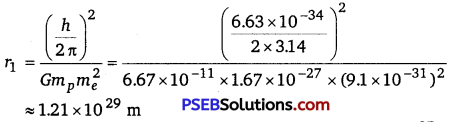Question 13.
Obtain an expression for the frequency of radiation emitted when a hydrogen atom de-excites from level n to level (n -1). For large n, show that this frequency equals the classical frequency of revolution of the electron in the orbit.
It is given that a hydrogen atom de-excites from an upper level (n) to a lower level (n —1). We have the relation for energy (E1) of radiation at level n as
E1 = hv1 = $$\frac{h m e^{4}}{(4 \pi)^{3} \varepsilon_{0}^{2}\left(\frac{h}{2 \pi}\right)^{3}} \times\left(\frac{1}{n^{2}}\right)$$
where, v1 = Frequency of radiation at level n
h = Planck’s constant
m = Mass of hydrogen atom
e = Charge on an electron
εo = Permittivity of free space

Now, the relation for energy (E2) of radiation at level (n -1) is given as
E2 = hv2 = $$\frac{h m e^{4}}{(4 \pi)^{3} \varepsilon_{0}^{2}\left(\frac{h}{2 \pi}\right)^{3}} \times \frac{1}{(n-1)^{2}}$$ ………………………… (2)
where, v2 = Frequency of radiation at level (n -1)
Energy (E) released as a result of de-excitation
E = E2 – E1 hv= E2 – E 1 ………………….. (3)
where, v = Frequency of radiation emitted
Putting values from equations (1) and (2) in equation (3), we get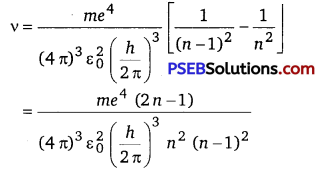For large n, we can write (2 n -1) ≈ 2 n and (n-1) ≈ n.
V = $$\frac{m e^{4}}{32 \pi^{3} \varepsilon_{0}^{2}\left(\frac{h}{2 \pi}\right)^{3} n^{3}}$$
∵ v = $$\frac{m e^{4}}{32 \pi^{3} \varepsilon_{0}^{2}\left(\frac{h}{2 \pi}\right)^{3} n^{3}}$$ ………………….. (4)
Classical relation of frequency of revolution of an electron is given as
Vc = $$\frac{v}{2 \pi r}$$ ……………………………….. (5)
where, velocity of the electron in the nth orbit is given as
v = $$\frac{e^{2}}{4 \pi \varepsilon_{0}\left(\frac{h}{2 \pi}\right) n}$$ ……………………………… (5)
And, radius of the nth orbit is given as
v = $$\frac{e^{2}}{4 \pi \varepsilon_{0}\left(\frac{h}{2 \pi}\right) n}$$ ………………………………(6)
Putting the values of equations (6) and (7) in equation (5), we get
Vc = $$\frac{m e^{4}}{32 \pi^{3} \varepsilon_{0}^{2}\left(\frac{h}{2 \pi}\right)^{3} n^{3}}$$
Hence, the frequency of radiation emitted by the hydrogen atom is equal to its classical orbital frequency.

Question 14.
Classically, an electron can be in any orbit around the nucleus of an atom. Then what determines the typical atomic size? Why is an atom not, say, a thousand times bigger than its typical size? The question had greatly puzzled Bohr before he arrived at his famous model of the atom that you have learnt in the text.

To simulate what he might well have done before his discovery, let us play as follows with the basic constants of nature and see if we can get a quantity with the dimensions of length that is roughly equal to the known size of an atom (~10-10 m).

(a) Construct a quantity with the dimensions of length from the fundamental constants e, me and c. Determine its numerical value.

(b) You will find that the length obtained in (a) is many orders of magnitude smaller than the atomic dimensions. Further, it involves c. But energies of atoms are mostly in non-relativistic domain where c is not expected to play any role. This is what may have suggested Bohr to discard c and look for ‘something else’ to get the right atomic size. Now, the Planck’s constant h had already made its appearance elsewhere. Bohr’s great insight lay in recognizing that h, me, and e will yield the right atomic size.

Construct a quantity with the dimension of length from h, me, and e and confirm that its numerical value has indeed the correct order of magnitude.
(a) Charge on an electron, e = 1.6 x 10-19 C
Mass of an electron, me = 9.1 x 10-31 kg
Speed of light, c = 3 x 108 m/s
Let us take a quantity involving the given quantities as $$\left(\frac{e^{2}}{4 \pi \varepsilon_{0} m_{e} c^{2}}\right)$$
where, ε0 = Permittivity of free space and, $$\frac{1}{4 \pi \varepsilon_{0}}$$ = 9 x 109 Nm2C-2 .
The numerical value of the taken quantity will be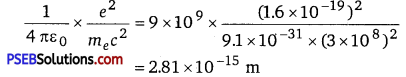Hence, the numerical value of the taken quantity is much smaller than the typical size of an atom.

(b) Charge on an electron, e = 1.6 x 10-19 C
Mass of an electron, me = 9.1 x 10-31 kg
Planck’s constant, h = 6.63 x 10-34 Js
Let us take a quantity involving the given quantities as $$\frac{4 \pi \varepsilon_{0}\left(\frac{h}{2 \pi}\right)^{2}}{m_{e} e^{2}}$$
where, ε0 = Permittivity of free space
and, $$\frac{1}{4 \pi \varepsilon_{0}}$$ = 9 x 109Nm2C-2

The numerical value of the taken quantity will be
$$\frac{1}{4 \pi \varepsilon_{0}} \times \frac{\left(\frac{h}{2 \pi}\right)^{2}}{m_{e} e^{2}}=9 \times 10^{9} \times \frac{\left(\frac{6.63 \times 10^{-34}}{2 \times 3.14}\right)^{2}}{9.1 \times 10^{-31} \times\left(1.6 \times 10^{-19}\right)^{2}}$$
= 0.53 x 10-10 m
Hence, the value of the quantity taken is of the order of the atomic size.Question 15.
The total energy of an electron in the first excited state of the hydrogen atom is about -3.4 eV.
(a) What is the kinetic energy of the electron in this state?
(b) What is the potential energy of the electron in this state?
(c) Which of the answers above would change if the choice of the zero of potential energy is changed?
(a) Total energy of the electron, E = -3.4 eV ’
Kinetic energy of the electron is equal to the negative of the total energy.
⇒ K = -E
= -(-3.4) = + 3.4 eV
Hence, the kinetic energy of the electron in the given state is + 3.4 eV.

(b) Potential energy (JJ) of the electron is equal to the negative of twice of its kinetic energy.
⇒ U = -2 K
= -2 x 3.4 = -6.8 eV
Hence, the potential energy of the electron in the given state is -6.8 eV.

(c) The potential energy of a system depends on the reference point taken. Here, the potential energy of the reference point is taken as zero. If the reference point is changed, then the value of the potential energy of the system also changes. Since total energy is the sum of kinetic and potential energies, total energy of the system will also change.

Question 16.
If Bohr’s quantization postulate (angular momentum = nh/2π) is a basic law of nature, it should be equally valid for the case of planetary motion also. Why then do we never speak of quantization of orbits of planets around the sun?
In Bohr’s atom model, r ∝ $$\frac{1}{m}$$
∵ $$\frac{r_{\text {muon }}}{r_{\text {electron }}}=\frac{m_{e}}{m_{\mu}}=\frac{m_{e}}{207 m_{e}}=\frac{1}{207}$$ [ ∵mμ = 207 me]